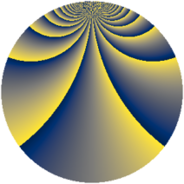# Properties

 Label 1210.2.xLevel $1210$ Weight $2$ Character orbit 1210.x Rep. character $\chi_{1210}(7,\cdot)$ Character field $\Q(\zeta_{220})$ Dimension $5280$ Sturm bound $396$

# Related objects

## Defining parameters

 Level: $$N$$ $$=$$ $$1210 = 2 \cdot 5 \cdot 11^{2}$$ Weight: $$k$$ $$=$$ $$2$$ Character orbit: $$[\chi]$$ $$=$$ 1210.x (of order $$220$$ and degree $$80$$) Character conductor: $$\operatorname{cond}(\chi)$$ $$=$$ $$605$$ Character field: $$\Q(\zeta_{220})$$ Sturm bound: $$396$$

## Dimensions

The following table gives the dimensions of various subspaces of $$M_{2}(1210, [\chi])$$.

Total New Old
Modular forms 16160 5280 10880
Cusp forms 15520 5280 10240
Eisenstein series 640 0 640

## Trace form

 $$5280q + 8q^{5} + 20q^{7} + O(q^{10})$$ $$5280q + 8q^{5} + 20q^{7} - 44q^{10} - 12q^{11} - 88q^{13} + 12q^{15} - 132q^{16} + 20q^{17} - 84q^{22} - 8q^{23} + 32q^{25} - 8q^{26} + 20q^{28} - 16q^{31} + 104q^{33} - 116q^{36} + 124q^{37} + 168q^{38} + 20q^{41} + 20q^{42} + 32q^{45} - 40q^{46} - 44q^{47} - 40q^{50} - 392q^{51} - 40q^{52} + 4q^{53} - 8q^{55} + 8q^{56} - 48q^{58} - 24q^{60} - 80q^{61} - 40q^{62} - 320q^{63} - 24q^{66} - 192q^{67} - 20q^{68} - 48q^{70} + 16q^{71} + 20q^{73} + 64q^{75} + 44q^{76} + 96q^{77} - 16q^{78} + 12q^{80} + 1360q^{81} - 16q^{82} - 96q^{85} + 56q^{86} + 4q^{88} + 80q^{90} + 332q^{91} - 36q^{92} - 76q^{93} - 20q^{95} - 60q^{97} + O(q^{100})$$

## Decomposition of $$S_{2}^{\mathrm{new}}(1210, [\chi])$$ into newform subspaces

The newforms in this space have not yet been added to the LMFDB.

## Decomposition of $$S_{2}^{\mathrm{old}}(1210, [\chi])$$ into lower level spaces

$$S_{2}^{\mathrm{old}}(1210, [\chi]) \cong$$ $$S_{2}^{\mathrm{new}}(605, [\chi])$$$$^{\oplus 2}$$